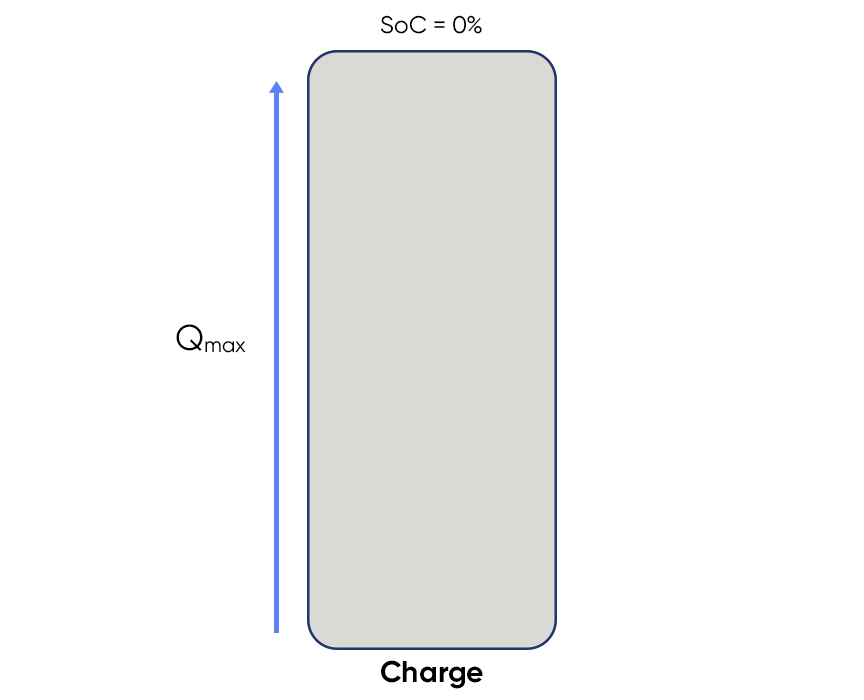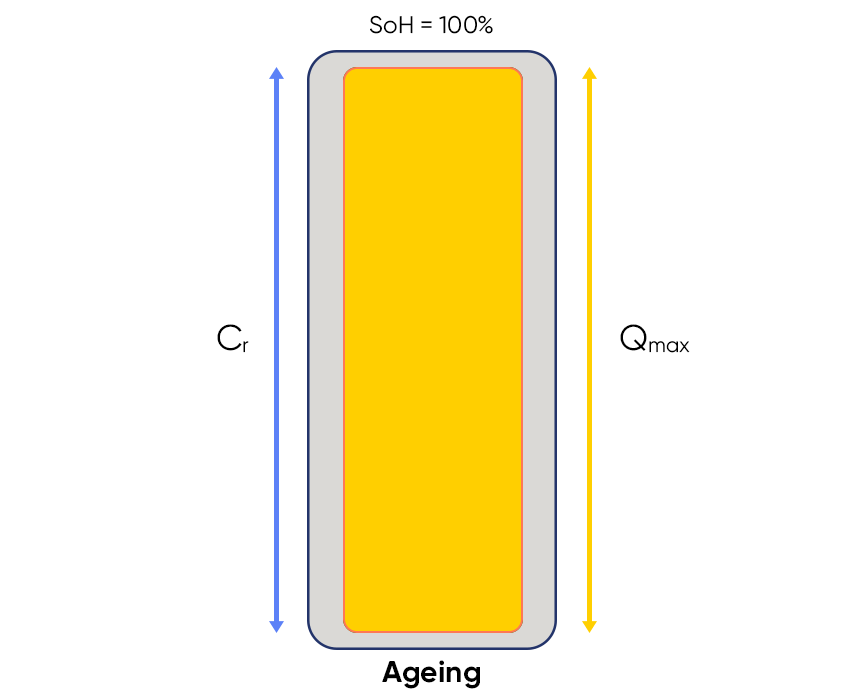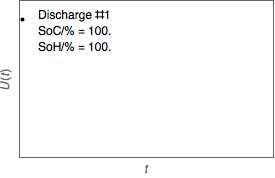Topic 5 min read# Battery states: State of charge (SoC), State of Health (SoH). Electrochemistry basics series.

Latest updated: June 13, 2023

## What are SoC (state of charge) and SoH (state of health) for a battery?

Understanding and monitoring cells’ states, at a particular point in time, is often needed in battery development in order to optimize their use.

You may want to better understand the State-of-Charge $\mathrm{(SoC)}$ and State-of-Health $\mathrm{(SoH)}$ of the battery. These parameters are important because they are directly related to battery performance.

State-of-health $\mathrm{(SoH)}$ and State-of-Charge $\mathrm{(SoC)}$ are key quality indicators as they provide very useful data needed for the optimization of the Battery Management System (BMS).

State-of-charge and state-of-health are different parameters that can sometimes be confused. The aim of this article is to clearly define each term and explain its value and use.

## $\mathbf{SoC}=$ State-of-charge

The state of charge of a battery describes the difference between a fully charged battery and the same battery in use. It is associated with the remaining quantity of electricity available in the cell.

It is defined as the ratio of the remaining charge in the battery, divided by the maximum charge that can be delivered by the battery. It is expressed as a percentage as below.

$$\mathrm{SoC/\%}=100\frac{(Q_0+Q)}{Q_{\mathrm{max}}}=\mathrm{SoC_0/\%}+100\frac{Q}{Q_{\mathrm{max}}} \tag{1}$$

$Q_0/\mathrm{mAh\;}=$ Initial charge of the battery.

$Q/\mathrm{mAh\;}=$ The quantity of electricity delivered by or supplied to, the battery. It follows the convention of the current: it is negative during the discharge and positive during the charge.

$Q_{\mathrm{max}}/\mathrm{mAh\;}=$  The maximum charge that can be stored in the battery.

$\mathrm{SoC_0/\%}=$ The initial state-of-charge $\mathrm{(SoC/\%)}$ of the battery.

• If the battery is new: $Q_{\mathrm{max}}=C_{\mathrm{r}}$ and $Q_0=0.5\,Q_{\mathrm{max}}$ generally. $C_{\mathrm{r}}$ is the rated capacity of the battery as given by the manufacturer.
• If the battery is fully charged: $Q_0=Q_{\mathrm{max}}$ and $\mathrm{SoC_0}=100\%$.Figure 1: Evolution of the $\mathbf{S_0C_0}$ of a battery during charge and discharge

The state-of-charge may also be considered the other way around and it is called the Depth of Discharge $\mathrm{(DoD)}$. It is calculated as followed:

$$\mathrm{DoD/\%}=100-\mathrm{SoC/\%}\tag{2}$$

## $\mathbf{SoH}=$ State-of-Health

The state-of-health (SoH of a battery describes the difference between a battery being studied and a fresh battery and considers cell aging.

It is defined as the ratio of the maximum battery charge to its rated capacity. It is expressed as a percentage as seen below.

$$\mathrm{SoH/\%}=100\frac{Q_{\mathrm{max}}}{C_{\mathrm{r}}}\tag{3}$$

$Q_{\mathrm{max}}/\mathrm{mAh\;}=$  The maximum charge available of the battery

$C_{\mathrm{r}}=$ The rated capacityFigure 2: Evolution of the $\mathbf{SoH}$  of a battery during aging

The discharge profile of a secondary battery is affected by its state of health. The lower the $\mathrm{SoH}$, the faster the battery is discharged as it is illustrated in the Figure 3 below.Figure 3: $\mathbf{U}$ vs. $\mathbf{t}$ during battery charge and discharge cycles for different $\mathbf{SoH}$

## How to measure $\mathbf{SoC}$ and/or $\mathbf{SoH}$ with a BioLogic potentiostat / galvanostat or battery cycler

The $\mathrm{SoC}$  value is reachable by monitoring the charge of the battery (measurement of the current and the time). To this end, Galvanostatic Cycling with Potential Limitation (GCPL) technique as well as Chronopotentiometry (CP) and Constant Current (CC) techniques are suitable.

One of the ways available of determining the $\mathrm{SoH}$ is to know the quantity of charge $Q$ that can be stored at a specific stage. Therefore, the EC-Lab® or BT-Lab® Battery Capacity Determination (BCD) technique can be used in this purpose.

State of charge State of health Battery Capacity Determination (BCD) Rated capacity GCPL (Galvanostatic Cycling with Potential Limit) Constant Current (CC) Chronopotentiometry (CP)

## Related accessories

Work smarter. Not harder.

Tech-tips, theory, latest functionality, new products & more.

No thanks!